## 5.16Preconditioning and asymmetry

The conjugate gradient (CG) method provides a good basis for a matrix solver in CFD. In theory, it provides an exact solution in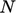steps for a problem withvalues. In practice, there is an accumulation of rounding errors from ﬁnite precision arithmetic, such that an exact solution is not attained. Problems are also too large for it to be feasible to runsteps.

CG is instead used as an iterative method, aiming to get the solution residual within an acceptable tolerance. The rate of convergence is therefore critical, which can be shown to be slower for increasing condition number of the matrix,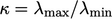, where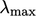and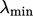are its largest eigenvalues.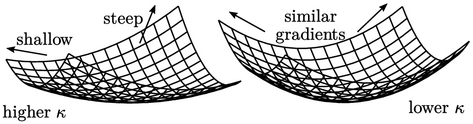In the example with two values, the eigenvalues correspond to the gradients of the principal axes of the paraboloid described by the quadratic function. Lower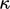corresponds to closer gradients, i.e. to a rounder “bowl” shape.

The best convergence corresponds to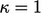when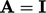(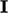is the identity matrix). Preconditioning ﬁnds a matrix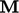, similar to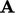, which can be inverted and multiplied to the equation as follows: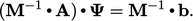(5.35)
The aim of preconditioning is to improve convergence by choosing a preconditioner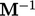so that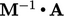is closer to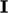than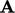. Eﬀective methods for calculating the preconditioner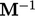are:
• diagonal-based incomplete Cholesky (DIC) for symmetric;
• diagonal-based incomplete LU (DILU) for an asymmetric.

The preconditioned CG (PCG) method minimises the quadratic form which requires thatis symmetric. However, any equation containing an advection term produces an asymmetric matrix (see Sec. 5.1 ), when PCG is unsuitable.

The preconditioned biconjugate gradient (PBiCG) method is designed for asymmetric matrices. It augments the calculations of residuals and line search directions in the CG method with a second set of calculations relating to the transpose matrix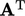. The computational cost is approximately 2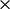the cost of PCG.

The convergence behaviour of PBiCG is somewhat erratic, with large variations in the reduction of the residual between successive iterations. Sometimes the method breaks down and fails.

The preconditioned biconjugate gradient stabilised method (PBiCGStab) is a modiﬁcation of PBiCG. It is exhibits much smoother convergence and robustness than PBiCG so it is far more preferable for CFD applications.

Recommended CG-based methods are summarised below.

• symmetric: PCG with DIC preconditioning.
• asymmetric: PBiCGStab with DILU preconditioning.

The implementation of preconditioning and CG-based methods can be found in: Barrett R. et al. Templates for the solution of linear systems, available at http://www.netlib.org.

Notes on CFD: General Principles - 5.16 Preconditioning and asymmetry